Worksheet: Chemical Reactions & Equations - 2

# Worksheet: Chemical Reactions & Equations - 2 - Science Class 10

Q.1.

Question for Worksheet: Chemical Reactions & Equations - 2
Try yourself:Which of the following reactions is an endothermic reaction?

The physical states of the reactants and products can be represented by using the symbols (s) for solids, (l) for liquids, (g) for gases, and (aq) for an aqueous solution along with their respective formulae. The word aqueous is written if the reactant or product is present as a water solution. Precipitation can also be represented using an arrow pointing downwards (↓) instead of using the symbol (s). Similarly, the gaseous state of an evolved gas can be represented by using an arrow pointing upward direction (↑) instead of using the symbol (g). The specific condition of the reaction, like temperature, pressure, catalyst, etc., is written above or below the arrow in the chemical equation.
(a) Write the balanced chemical equation with state symbols for the given reaction: Magnesium reacting with dilute sulphuric acid  [1 Marks]
(b) To indicate a gaseous reactant or product, we use the _____ or ____ symbol.  (1 Marks)
(c) Complete the missing variable given as X and Y in the following reaction:   (1 Marks)

2Na (s) + 2H2O (l) → 2NaOH (X) + H2 (Y)

(d) Which of the following reactions is balanced?  (1 Marks)

(i) 2NaCl + 2H2O → 2NaOH + 2Cl2 + H2

(ii) Ca(OH)2 + Cl2 → CaOCl2 + H2O
Ans.
(a) Mg (s) + H2SO4 (aq) → MgSO4 (aq) + H2
(b) (g) or ↑
(c) X is (aq) and Y is (g)
(d) Reaction II is balanced.

Q.3. (a) Write two observations when lead nitrate is heated in a test tube.   (1 Marks)
(b) Name the type of reaction.  (1 Marks)
(c) Write a balanced chemical equation to represent the above reaction.  (1 Marks)

Ans. (a) It turns yellow due to lead oxide and reddish-brown fumes' formation.
(b) Thermal decomposition reaction.
(c) (CBSE Marking Scheme, 2020)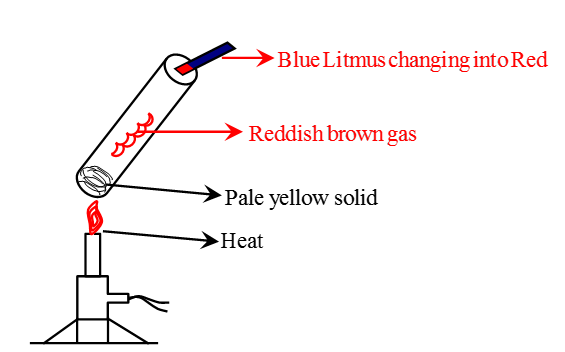Observation after heating Lead Nitrate in a test tubeQ.4. Define a chemical reaction. State four observations help us determine that a chemical reaction has taken place. Write one example of each observation with a balanced chemical equation.  (5 Marks)
Ans.
Process in which the rearrangement of atoms forms new substances with new properties.
(i) Evolution of gas: The chemical reaction between zinc and dilute H2SO4.

Zn(s) + H2SO4(aq) → ZnSO4(aq) + H2(g) ↑
(ii) Change in color: The chemical reaction between potassium iodide and lead nitrate.

Pb(NO3)2(aq) + 2KI(s) → 2KNO3(aq) + PbI2(s)

Colorless Yellow
(iii) Formation of precipitate: The chemical reaction between sulphuric acid and barium chloride.

BaCl2(aq) + H2SO4(aq) → 2HCl(aq) + BaSO4(s)

White precipitate
(iv) Change in temperature: The chemical reaction between quicklime and water.
CaO(s) + H2O (l) → Ca(OH)2(aq) + Heat

Q.5.

Question for Worksheet: Chemical Reactions & Equations - 2
Try yourself:Which one of the following processes involves chemical reactions?

Q.6. Why is hydrogen peroxide kept in colored bottles? (1 Marks)
Ans. Hydrogen peroxide decomposes into H2O and O2 in the presence of sunlight, and hence to prevent decomposition, they are kept in colored bottles.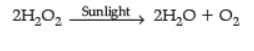Q.7. 2 g of silver chloride is taken in a china dish, and the china dish is placed in sunlight for some time. What will be your observation in this case? Write the chemical reaction involved in the form of a balanced chemical equation. Identify the type of chemical reaction. (3 Marks)
Ans.

• White silver chloride turns grey in sunlight.
•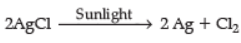• Decomposition reaction/Photolytic decomposition. (CBSE Marking Scheme, 2019)

Q.8. (i) Define corrosion.
(ii) What is corrosion of iron called?
(iii) How will you recognize the corrosion of silver?
(iv) Why is iron corrosion a serious problem?
(v) How can we prevent corrosion of iron?  (1 Marks)

Ans. (i) Corrosion is a process in which metals are deteriorated by air, moisture, chemicals, etc.
(ii) Rusting.
(iii) Silver - black, copper green.
(iv) It destroys car bodies, bridges, railing, etc.  (Any two)
(v) By Painting, alloying, greasing, etc.  (Any two)

(i) Corrosion is a process in which metals are deteriorated by the action of air, moisture, chemicals, etc.
(ii) Corrosion of iron is called rusting.
(iii) Silver turns black as it reacts with H2S present in the air and forms a layer of Ag2S.
(iv) Corrosion of iron is a serious problem because it leads to the wastage of tonnes of iron every year, and a lot of money is spent to repair or replace it.
(v) The iron articles should be painted to prevent corrosion. (CBSE Marking Scheme, 2016)

Q.9. The following reaction is an example of a

4NH3(g) + 5O2(g) → 4NO(g) + 6H2O(g)
(i) Displacement reaction
(ii) Combination reaction
(iii) Redox reaction
(iv) Neutralisation reaction  (1 Marks)
(a)
(i) and (iv)
(b) (ii) and (iii)
(c) (i) and (iii)
(d) (iii) and (iv)
Ans. (c)
Solution. The given reaction is a redox reaction because oxidation and reduction both take place simultaneously. Also, it is a displacement reaction because the hydrogen of NH3 has been displaced by oxygen.

P, Q, and R are 3 elements that undergo chemical reactions according to the following equations:

(i) P2O3 + 2Q → Q2O3 + 2P
(ii) 3RSO4 + 2Q → Q2(SO4)3 + 3R
(iii) 3RO + 2P → P2O3 + 3R
(a) Which element is most reactive? (1 Marks)
(b) Which element is least reactive? (1 Marks)
(c) The type of reactions is (1 Marks)

(i) Displacement reaction
(ii) Combination reaction
(iii) Neutralisation reaction
(iv) Substitution reaction
(d) Define the reaction.  (1 Marks)
Ans. (a) Q, as it has replaced both P and R from their compounds
(b) R, as it has been replaced by both P and Q
(c) (i) Displacement reaction
(d) Displacement reaction is a reaction in which a more active element displaces a less reactive element from its compound.

Q.11. N2 + 3H2 → 2NH3, name the type of reaction. (1 Marks)
Ans. It is a combination reaction.

Q.12. On heating blue colored powder of copper (ii) nitrate in a boiling tube, black copper oxide, O2, and a brown gas X are formed.
(a) Identify the type of reaction and the gas X.
(b) Write a balanced chemical equation of the reaction.
(c) Write the pH range of the aqueous solution of the gas X.  (3 Marks)

Ans. (a) Decomposition / Thermal decomposition,

The gas X is NO2 or (nitrogen dioxide)
(b)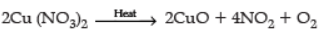(c) Range less than 7 (or 0------6.9pH)  (CBSE Marking Scheme, 2019)

(a) Type of reaction: Thermal decomposition reaction. The gas X is nitrogen dioxide.
(b) 2Cu(NO3)2(s) → 2CuO(s) + 4NO2(g) + O2(g)

Copper nitrate(II)        Black       Brown
(c) As oxides of non-metals are acidic in nature, an aqueous solution of nitrogen dioxide will have a pH range between 0 and 7.

Q.13. During the reaction of some metals with dilute hydrochloric acid, the following observations were made by a change.
(a) Silver does not show any change.
(b) Some bubbles of gas are seen when the lead is reacted with the acid.
(c) The reaction of sodium is found to be highly explosive.
(d) The reaction mixture's temperature rises when aluminum is added to the acid.

Explain these observations giving appropriate reasons.  (3 Marks)
Ans. (a) Silver is placed below Hydrogen in reactivity series / among least reactive metal / Silver does not react with dil. Hydrochloric acid.
(b) Rate of reaction is slow / bubbles of Hydrogen gas are formed / lead lies above hydrogen in reactivity series.
(c) Sodium is highly reactive / reaction is highly exothermic, evolving Hydrogen gas, which catches fire.
(d) Reaction is exothermic

(a) As silver is less reactive, it does not react with dilute hydrochloric acid.
(b) Bubbles are seen due to the evolution of hydrogen gas.

Pb(s) + 2HCl (aq) → PbCl2(aq) + H2(g)
(c) As sodium is a highly reactive metal, it reacts with dilute hydrochloric acid vigorously with heat evolution.
(d) The reaction between aluminum with dilute hydrochloric acid is exothermic; thus, the mixture's temperature rises with the addition of aluminum. (CBSE Marking Scheme, 2019)

Q.14.

Question for Worksheet: Chemical Reactions & Equations - 2
Try yourself:The following reaction is an example of a

4NH3(g) + 5O2(g) → 4NO(g) + 6H2O(g)
(i) Displacement reaction
(ii) Combination reaction
(iii) Redox reaction
(iv) Neutralisation reaction

Q.15. Assertion and Reason:
Directions: In the following questions, a statement of assertion (a) is followed by a reason (R). Mark the correct choice as :
(i)
Question for Worksheet: Chemical Reactions & Equations - 2
Try yourself:Assertion (A): Sodium metal is stored under Kerosene.
Reason (R): Metallic sodium melts when exposed to air.

(ii)

Question for Worksheet: Chemical Reactions & Equations - 2
Try yourself:Assertion (A): To dilute sulphuric acid, acid is added to water and not water to acid.
Reason (R): Specific heat of water is quite large.

Q.16. Decomposition reactions require energy either in heat or light or electricity for breaking down the reactants. Write one equation for decomposition reactions where energy is supplied in the form of heat, light, and electricity.  (3 Marks)
Ans.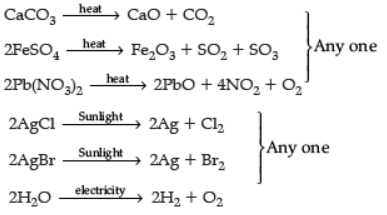(or any other equation for the above decomposition reaction.)
Note: No marks to be deducted if equations are not balanced.  (CBSE Marking Scheme, 2018)

Q.17. In the reaction :
MnO2 + 4HCl → MnCl2 + 2H2O + Cl2
(a) Name the compound (i) oxidized, (ii) reduced.
(b) Define oxidation and reduction on its basis. (3 Marks)

Ans. (a) (i) HCl is oxidized.
(ii) MnO2 is reduced.
(b) (i) Oxidation: Gain of Oxygen or loss of Hydrogen.
(ii) Reduction: Gain of Hydrogen or loss of Oxygen. (CBSE Marking Scheme, 2018)

Q.18. Why do silver articles become black after some time when exposed to air?  (1 Marks)
Ans.
They get tarnished by reacting with atmospheric air to form silver sulphide.

Q.19. Give the reason why chip manufacturers usually flush bags of chips with a gas such as nitrogen?  (1 Marks)
Ans. To prevent the chips' oil and fats from being oxidized or become rancid.

Q.20. Name the term used to indicate the development of unpleasant smell and taste in fats and oils containing food due to oxidation. What are antioxidants? Why are they added to fat and oil-containing food? (3 Marks)
Ans. Rancidity: Antioxidants are substances that prevent oxidation, are actually reducing agents. When added to food, the fats and oils present in the food do not get oxidized easily. Hence they do not turn rancid and remain good to eat for a longer time.

The document Worksheet: Chemical Reactions & Equations - 2 | Science Class 10 is a part of the Class 10 Course Science Class 10.
All you need of Class 10 at this link: Class 10

## Science Class 10

78 videos|509 docs|153 tests

## FAQs on Worksheet: Chemical Reactions & Equations - 2 - Science Class 10

 1. What is a chemical reaction?Ans. A chemical reaction is a process that involves the transformation of one or more substances into different substances. It occurs when chemical bonds are broken and new bonds are formed. This process is accompanied by the rearrangement of atoms and the release or absorption of energy.
 2. How can we represent a chemical reaction?Ans. A chemical reaction can be represented using a chemical equation. In a chemical equation, the reactants are written on the left side of the equation, and the products are written on the right side. The reactants are separated from the products by an arrow, indicating the direction of the reaction. Coefficients are used to balance the equation, ensuring that the number of atoms of each element is the same on both sides.
 3. What are the different types of chemical reactions?Ans. There are several types of chemical reactions, including: 1. Combination or synthesis reactions: These reactions occur when two or more substances combine to form a new compound. 2. Decomposition reactions: These reactions involve the breakdown of a compound into simpler substances. 3. Displacement or substitution reactions: In these reactions, one element replaces another element in a compound. 4. Double displacement or metathesis reactions: These reactions involve the exchange of ions between two compounds, resulting in the formation of two new compounds. 5. Redox reactions: Also known as oxidation-reduction reactions, these involve the transfer of electrons between reactants, resulting in changes in their oxidation states.
 4. How can you determine if a chemical reaction has occurred?Ans. There are several signs that indicate a chemical reaction has occurred: 1. Formation of a precipitate: A solid substance that forms when two solutions are mixed together. 2. Evolution of gas: Production of bubbles or a change in the volume of a gas. 3. Change in color: A noticeable color change in the reactants or products. 4. Release or absorption of heat: A change in temperature, either an increase or decrease, during the reaction. 5. Formation of a new substance: The formation of a compound with different properties than the reactants.
 5. Why is it important to balance chemical equations?Ans. It is important to balance chemical equations because they must follow the law of conservation of mass. This law states that matter cannot be created or destroyed in a chemical reaction, only rearranged. Balancing the equation ensures that the number of atoms of each element is the same on both sides, maintaining the overall mass of the system. Without balancing, the equation would not accurately represent the chemical reaction.

## Science Class 10

78 videos|509 docs|153 testsExplore Courses for Class 10 examSignup to see your scores go up within 7 days! Learn & Practice with 1000+ FREE Notes, Videos & Tests.
10M+ students study on EduRev
Track your progress, build streaks, highlight & save important lessons and more!
Related Searches

,

,

,

,

,

,

,

,

,

,

,

,

,

,

,

,

,

,

,

,

,

;